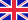•# Fuzzy Finite Element Method For Steady State Heat Conduction Problem

Autor: Sarangam Majumdar

Traditional finite element method is a well-established method to solve various problems of science and engineering. Different authors have used various methods to solve governing differential equation of heat conduction problem. In this study, heat conduction... Viac o knihe

Na objednávku, dodanie 2-4 týždne

42.84 €

bežná cena: 50.40 €

## O knihe

Traditional finite element method is a well-established method to solve various problems of science and engineering. Different authors have used various methods to solve governing differential equation of heat conduction problem. In this study, heat conduction in a circular rod has been considered which is made up of two different materials viz. aluminum and copper. In earlier studies parameters in the differential equation have been taken as fixed numbers which actually may not. Those parameters are found in general by some measurements or experiments. So the material properties are actually uncertain and may be considered to vary in an interval or as fuzzy and in that case complex interval arithmetic or fuzzy arithmetic has to be considered in the analysis.Hence interval/fuzzy arithmetic is applied in the finite element method to solve a steady state heat conduction problem. Application of fuzzy finite element method in the said problem gives fuzzy system of linear equations in general. Here we have also proposed new methods to handle such type of fuzzy system of linear equations. Corresponding results are computed and has been reported here.

• Vydavateľstvo: LAP LAMBERT Academic Publishing
• Rok vydania: 2012
• Formát: Paperback
• Rozmer: 220 x 150 mm
• Jazyk: Anglický jazyk
• ISBN: 9783659230349

Generuje redakčný systém BUXUS CMS spoločnosti ui42.